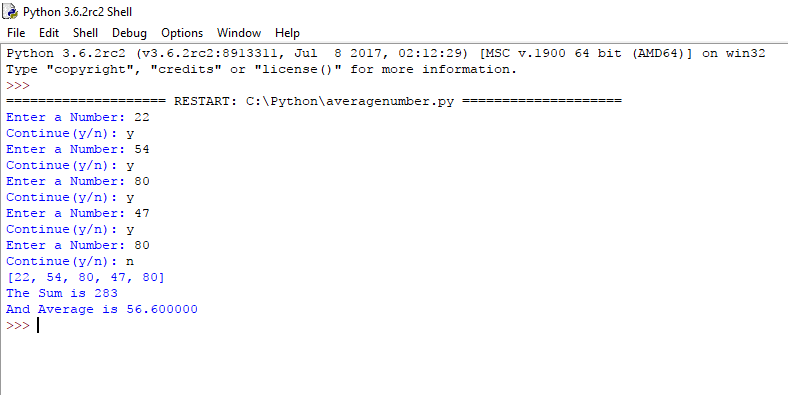# Python Basics : How To Calculate Average Number

This simple Python program allows the user to enter infinite values (with y/n condition ) and then add those entered numbers and displays the average number.

Python Code :-

```a=[ ]
b='y'

while b=='y':
n=input('Enter a Number: ')
a.append(int(n))
b=input('Continue(y/n): ')

s=0
for number in a:
s += number

avg=s/len(a)

print(a)
print("The Sum is %d" % (s))
print("And Average is %f" % (avg))```

Output :-